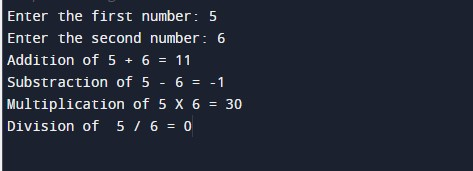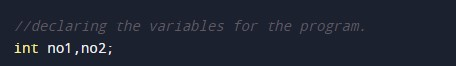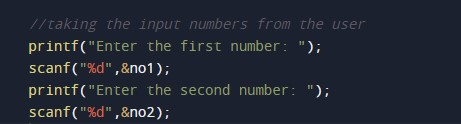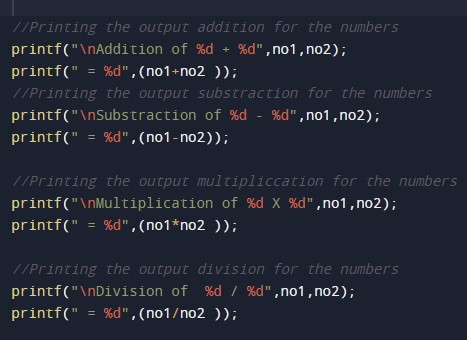C Program to Add Subtract Multiply Divide

In this tutorial you will learn about the C Program to Add Subtract Multiply Divide and its application with practical example.

C Program to Add Subtract Multiply Divide

In this tutorial, we will learn to create a C program that will Add, Subtract, Multiply & Divide using C programming.

Prerequisites

Before starting with this tutorial we assume that you are best aware of the following C programming topics:

• Operators in C Programming.
• Basic Input and Output function in C Programming.
• Basic C programming.
• Arithmetic operations in C Programming.

Program to Add Subtract Multiply Divide

In c programming, it is possible to take numerical input from the user and Add, Subtract, Multiply & Divide with the help of a very small amount of code. The C language has many types of header libraries which has supported function in them with the help of these files the programming is easy.

With the help of this program, we can Add Subtract Multiply Divide.

Here,

We will first take the input

The second will make a series and add the numbers.

Output:-In the above program, we have first initialized the required variable.• no1 = it will hold the integer value.
• no2 = it will hold the integer value.

Input number from the user.Program Logic Code and print the output.In this tutorial we have learn about the C Program to Add Subtract Multiply Divide and its application with practical example. I hope you will like this tutorial.